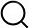# Also in the Article

Statistics and reproducibility

Procedure

Non-normal distribution of plating efficiency data was determined by Shapiro-Wilk test. Statistical significance for plating efficiency was calculated by Kruskal-Wallis one-way analysis of variance (ANOVA), followed by an ad hoc pairwise Wilcoxon rank sum test (Fig. 1B) from three biological replicates of three technical replicates. Statistical significance of exogenous CadA rescue (Fig. 1C) was calculated by Wilcoxon rank sum test. Non-normal distribution of saccharide inhibition data was determined by Shapiro-Wilk test. Statistical significance of saccharide inhibition of CadA binding (Fig. 3B) was determined by Wilcoxon rank sum test. Chemotactic index data represents n > 40 cells in each condition and was non-normally distributed by Shapiro-Wilk test (Fig. 5C). Significance for chemotactic indices was determined by Wilcoxon rank sum test (Fig. 5C). Non-normal distribution of speed measurements was determined by visual inspection of quantile-quantile plots and histograms and statistical significance was determined by Wilcoxon rank sum test (fig. S4A). CellROX-positive statistics were calculated using a two-tailed Student t test (Fig. 2B). Path length was determined to be normally distributed by Shapiro-Wilk test and statistical significance was determined by Welch’s t test for unequal variance (fig. S4B).

Q&A